Скачать презентацию Theory of Computation CS 302 Spring 2009

532320e74832381bde0126f4c0b44d11.ppt

• Количество слайдов: 63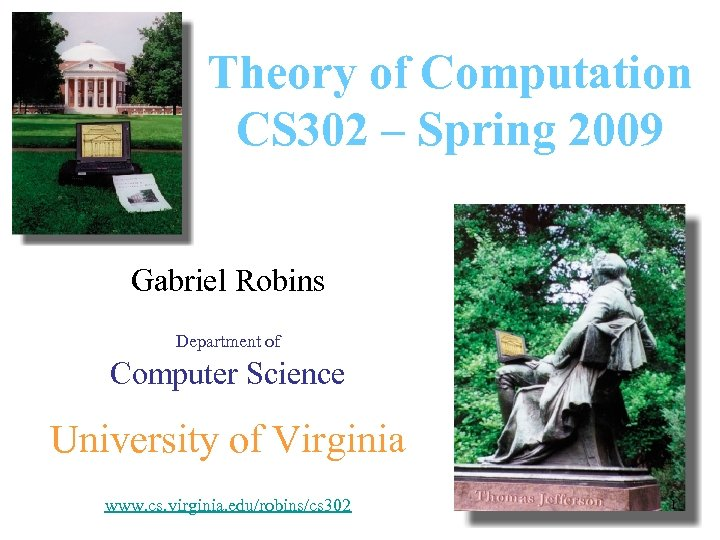Theory of Computation CS 302 – Spring 2009 Gabriel Robins Department of Computer Science University of Virginia www. cs. virginia. edu/robins/cs 302 1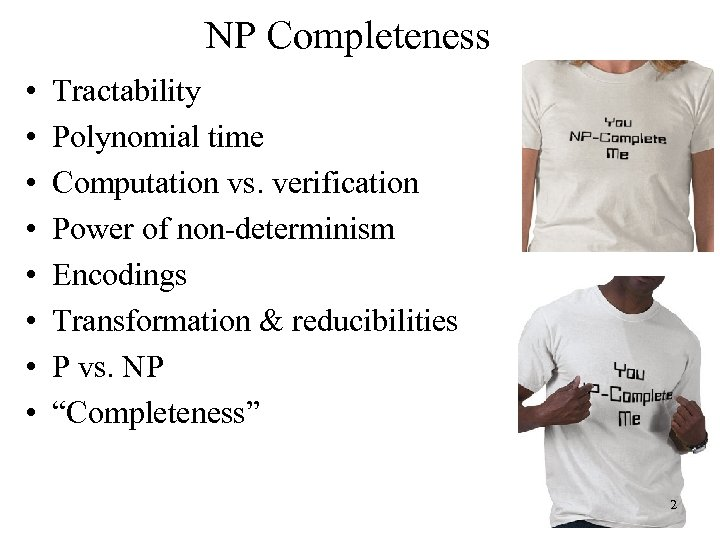NP Completeness • • Tractability Polynomial time Computation vs. verification Power of non-determinism Encodings Transformation & reducibilities P vs. NP “Completeness” 2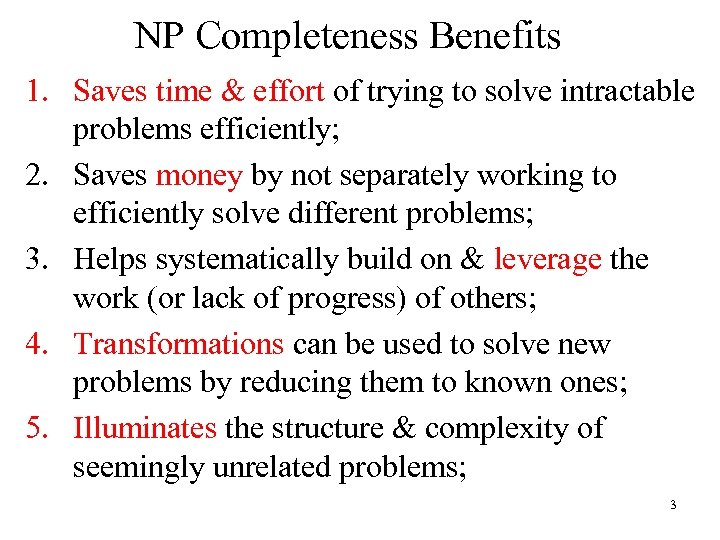NP Completeness Benefits 1. Saves time & effort of trying to solve intractable problems efficiently; 2. Saves money by not separately working to efficiently solve different problems; 3. Helps systematically build on & leverage the work (or lack of progress) of others; 4. Transformations can be used to solve new problems by reducing them to known ones; 5. Illuminates the structure & complexity of seemingly unrelated problems; 3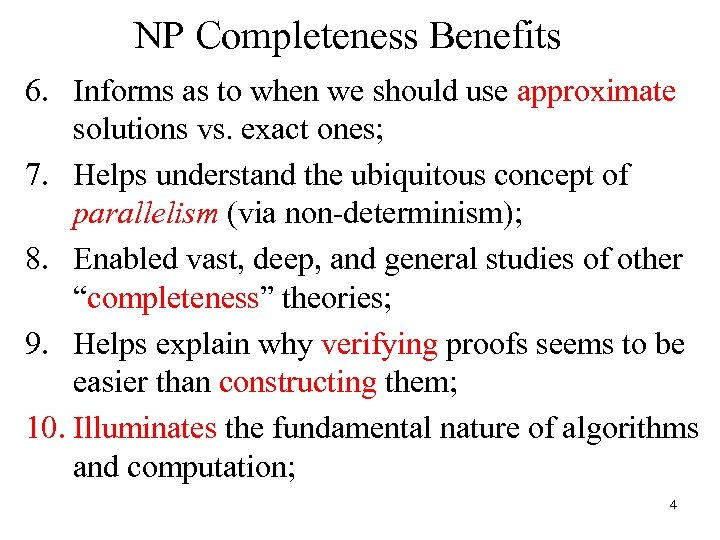NP Completeness Benefits 6. Informs as to when we should use approximate solutions vs. exact ones; 7. Helps understand the ubiquitous concept of parallelism (via non-determinism); 8. Enabled vast, deep, and general studies of other “completeness” theories; 9. Helps explain why verifying proofs seems to be easier than constructing them; 10. Illuminates the fundamental nature of algorithms and computation; 4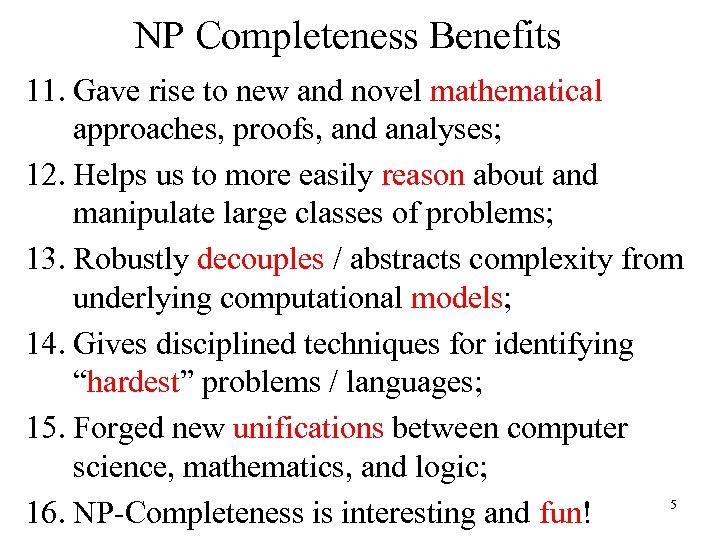NP Completeness Benefits 11. Gave rise to new and novel mathematical approaches, proofs, and analyses; 12. Helps us to more easily reason about and manipulate large classes of problems; 13. Robustly decouples / abstracts complexity from underlying computational models; 14. Gives disciplined techniques for identifying “hardest” problems / languages; 15. Forged new unifications between computer science, mathematics, and logic; 5 16. NP-Completeness is interesting and fun!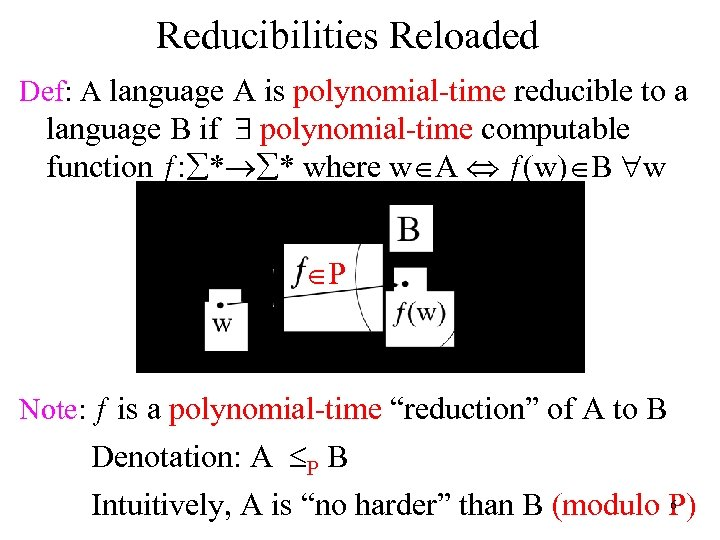Reducibilities Reloaded Def: A language A is polynomial-time reducible to a language B if \$ polynomial-time computable function ƒ: å*®å* where wÎA Û ƒ(w)ÎB "w ÎP Note: ƒ is a polynomial-time “reduction” of A to B Denotation: A £P B 6 Intuitively, A is “no harder” than B (modulo P)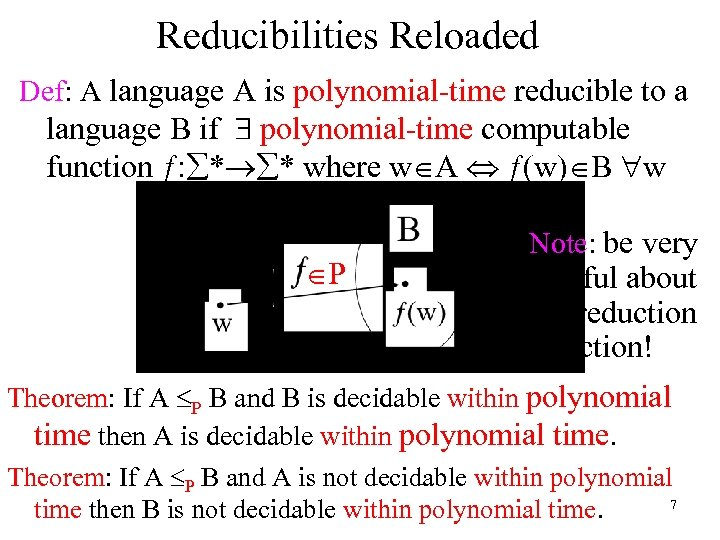Reducibilities Reloaded Def: A language A is polynomial-time reducible to a language B if \$ polynomial-time computable function ƒ: å*®å* where wÎA Û ƒ(w)ÎB "w ÎP Note: be very careful about the reduction direction! Theorem: If A £P B and B is decidable within polynomial time then A is decidable within polynomial time. Theorem: If A £P B and A is not decidable within polynomial 7 time then B is not decidable within polynomial time.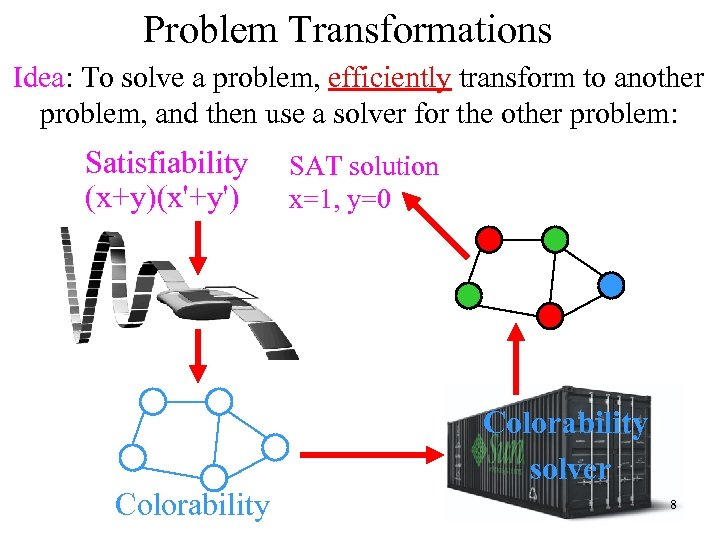Problem Transformations Idea: To solve a problem, efficiently transform to another problem, and then use a solver for the other problem: Satisfiability (x+y)(x'+y') Colorability SAT solution x=1, y=0 Colorability solver 8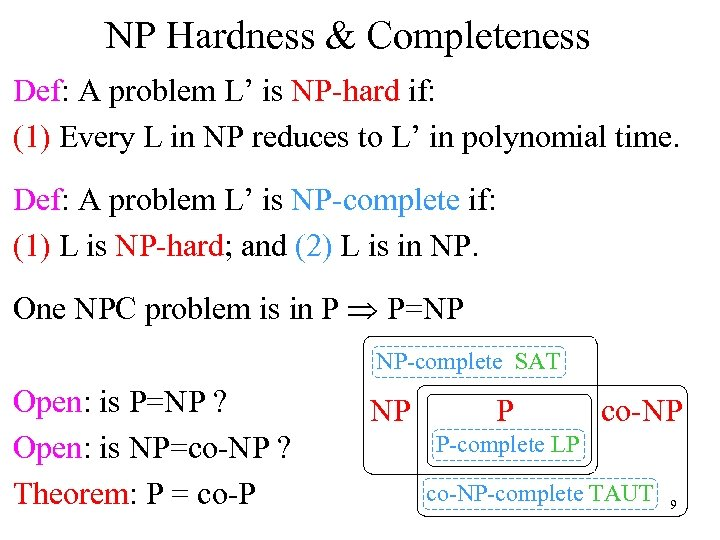NP Hardness & Completeness Def: A problem L’ is NP-hard if: (1) Every L in NP reduces to L’ in polynomial time. Def: A problem L’ is NP-complete if: (1) L is NP-hard; and (2) L is in NP. One NPC problem is in P Þ P=NP NP-complete SAT Open: is P=NP ? Open: is NP=co-NP ? Theorem: P = co-P NP P co-NP P-complete LP co-NP-complete TAUT 9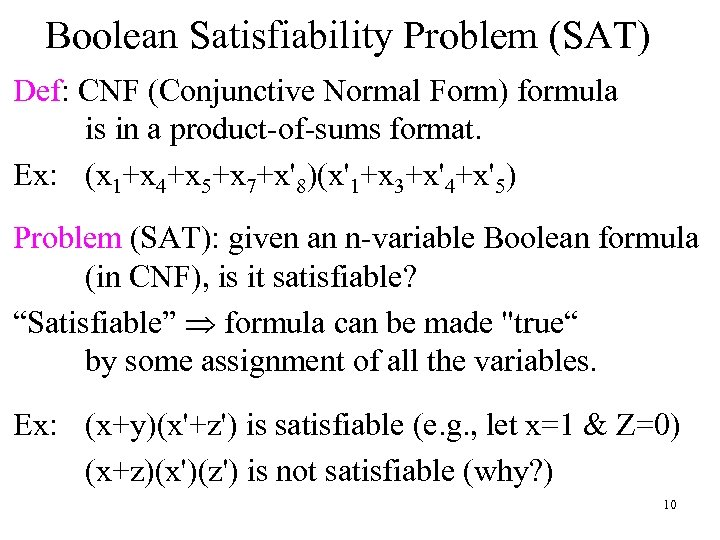Boolean Satisfiability Problem (SAT) Def: CNF (Conjunctive Normal Form) formula is in a product-of-sums format. Ex: (x 1+x 4+x 5+x 7+x'8)(x'1+x 3+x'4+x'5) Problem (SAT): given an n-variable Boolean formula (in CNF), is it satisfiable? “Satisfiable” Þ formula can be made "true“ by some assignment of all the variables. Ex: (x+y)(x'+z') is satisfiable (e. g. , let x=1 & Z=0) (x+z)(x')(z') is not satisfiable (why? ) 10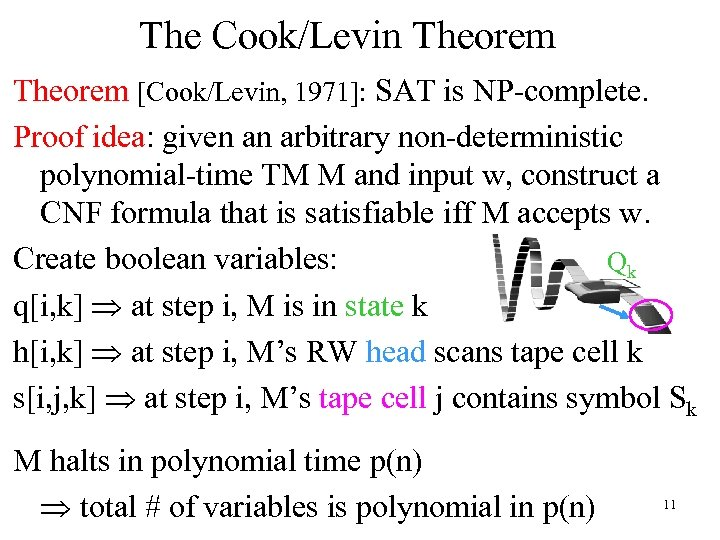The Cook/Levin Theorem [Cook/Levin, 1971]: SAT is NP-complete. Proof idea: given an arbitrary non-deterministic polynomial-time TM M and input w, construct a CNF formula that is satisfiable iff M accepts w. Create boolean variables: Qk q[i, k] Þ at step i, M is in state k h[i, k] Þ at step i, M’s RW head scans tape cell k s[i, j, k] Þ at step i, M’s tape cell j contains symbol Sk M halts in polynomial time p(n) Þ total # of variables is polynomial in p(n) 11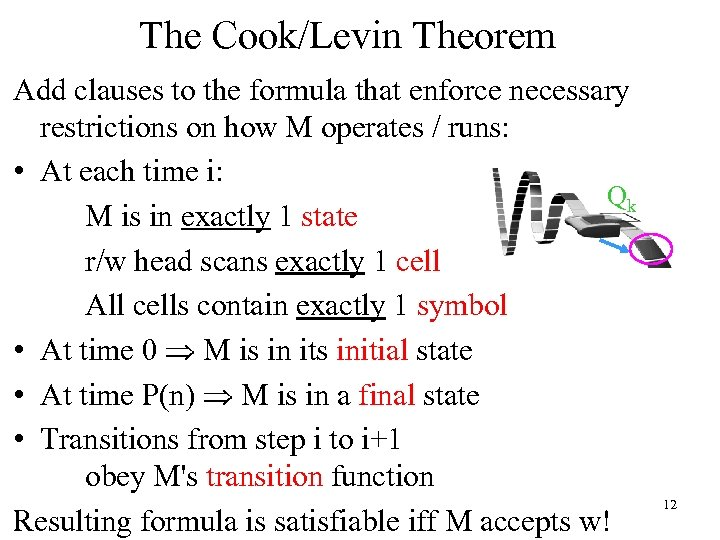The Cook/Levin Theorem Add clauses to the formula that enforce necessary restrictions on how M operates / runs: • At each time i: Qk M is in exactly 1 state r/w head scans exactly 1 cell All cells contain exactly 1 symbol • At time 0 Þ M is in its initial state • At time P(n) Þ M is in a final state • Transitions from step i to i+1 obey M's transition function Resulting formula is satisfiable iff M accepts w! 12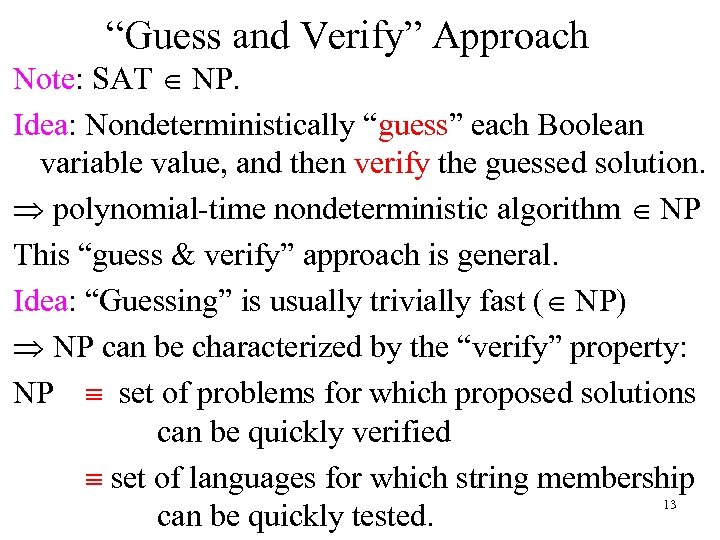“Guess and Verify” Approach Note: SAT Î NP. Idea: Nondeterministically “guess” each Boolean variable value, and then verify the guessed solution. Þ polynomial-time nondeterministic algorithm Î NP This “guess & verify” approach is general. Idea: “Guessing” is usually trivially fast (Î NP) Þ NP can be characterized by the “verify” property: NP º set of problems for which proposed solutions can be quickly verified º set of languages for which string membership 13 can be quickly tested.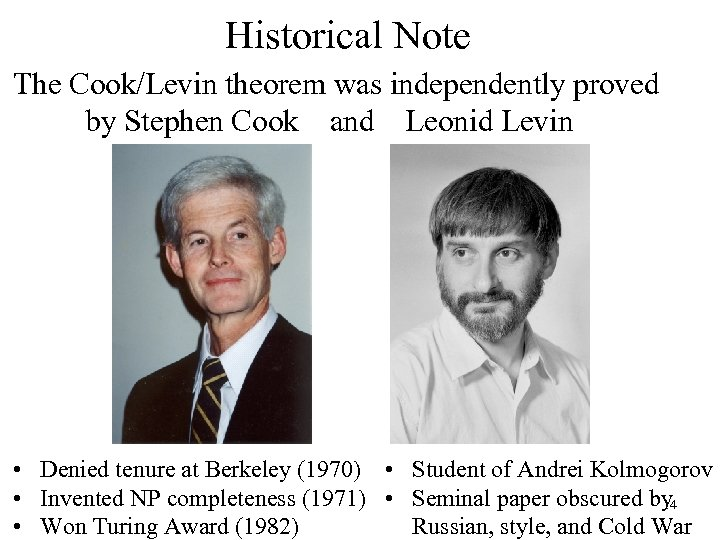Historical Note The Cook/Levin theorem was independently proved by Stephen Cook and Leonid Levin • Denied tenure at Berkeley (1970) • Student of Andrei Kolmogorov • Invented NP completeness (1971) • Seminal paper obscured by 14 • Won Turing Award (1982) Russian, style, and Cold War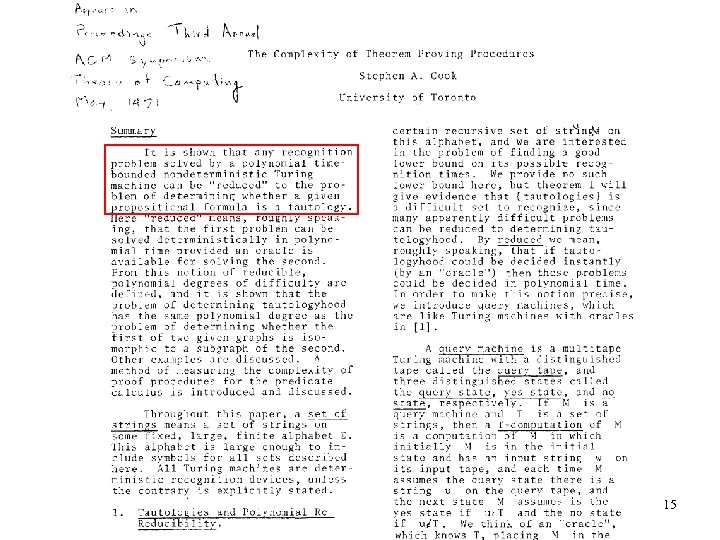15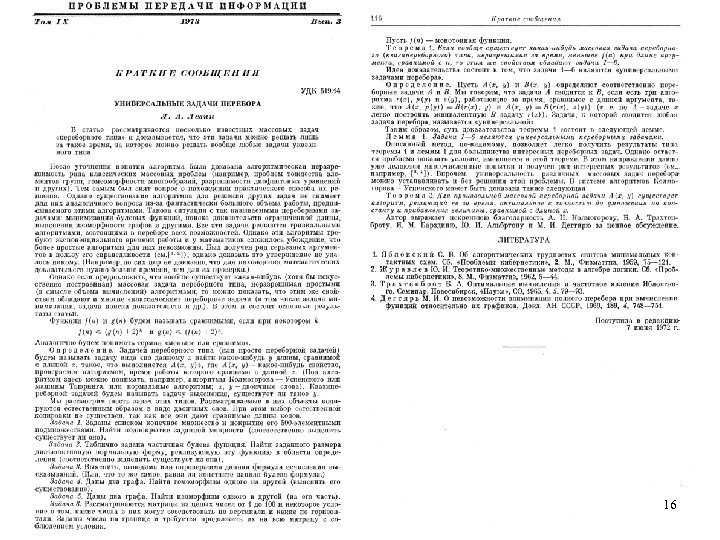16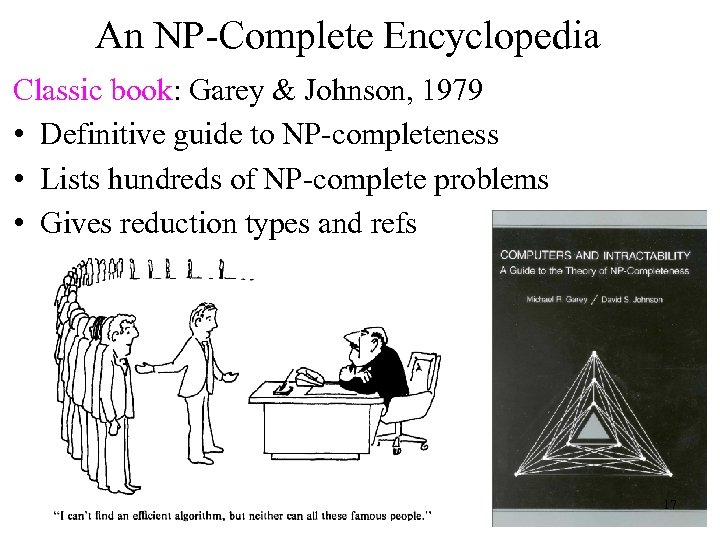An NP-Complete Encyclopedia Classic book: Garey & Johnson, 1979 • Definitive guide to NP-completeness • Lists hundreds of NP-complete problems • Gives reduction types and refs 17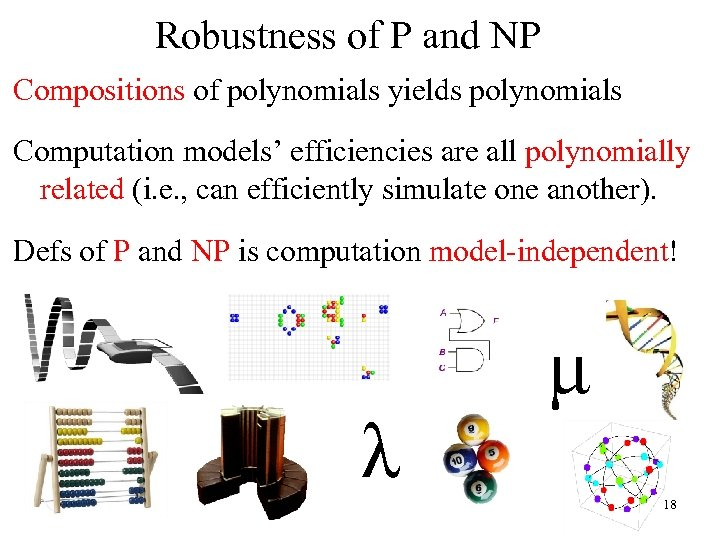Robustness of P and NP Compositions of polynomials yields polynomials Computation models’ efficiencies are all polynomially related (i. e. , can efficiently simulate one another). Defs of P and NP is computation model-independent! λ μ 18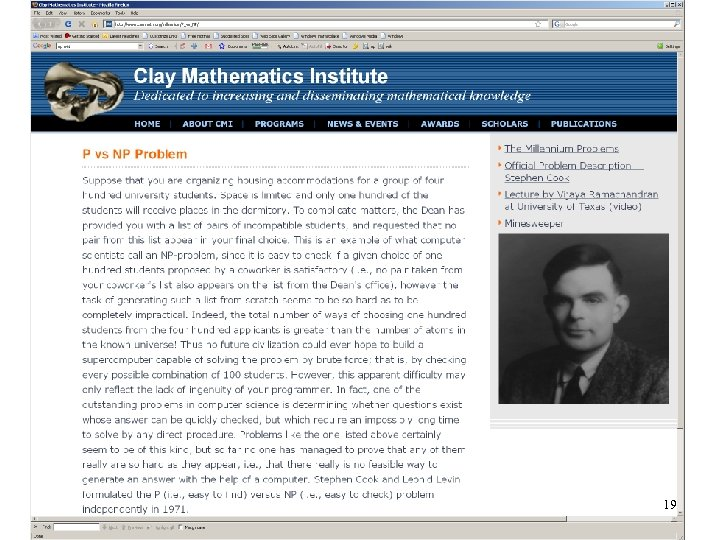19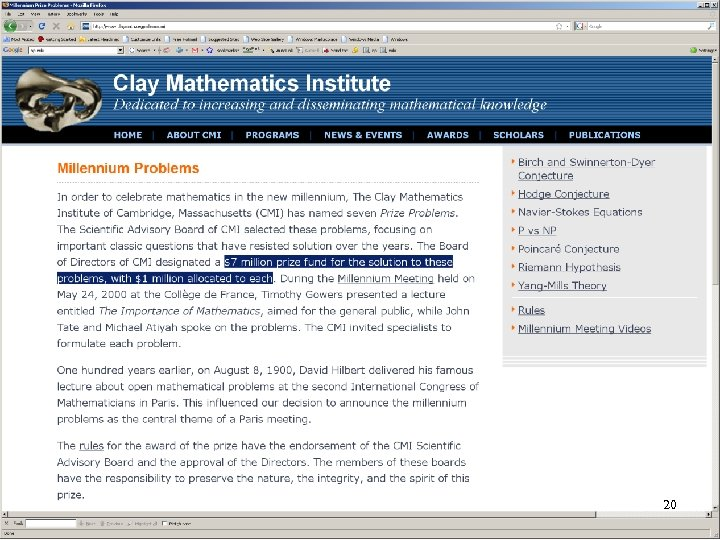20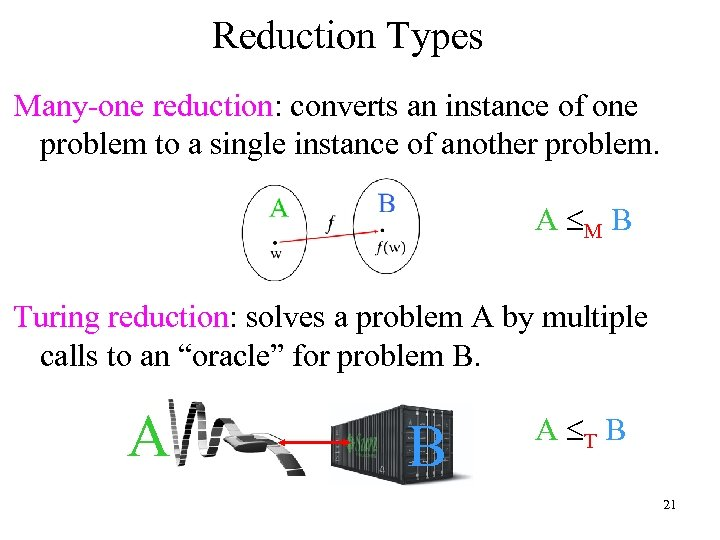Reduction Types Many-one reduction: converts an instance of one problem to a single instance of another problem. A £M B Turing reduction: solves a problem A by multiple calls to an “oracle” for problem B. A B A £T B 21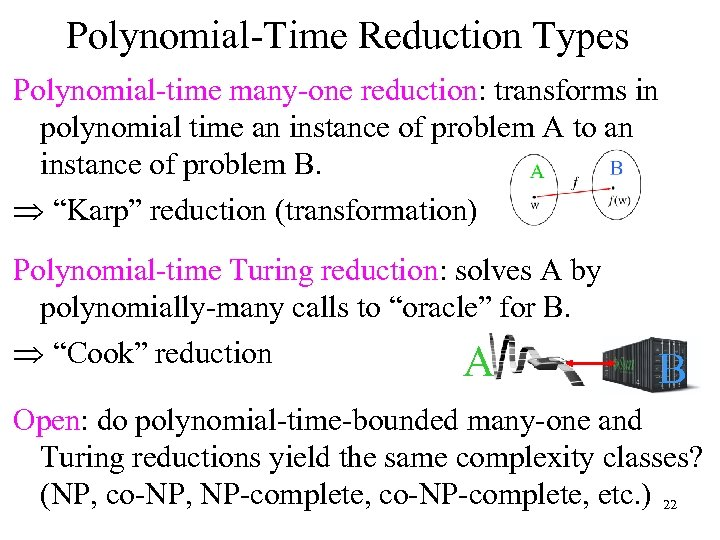Polynomial-Time Reduction Types Polynomial-time many-one reduction: transforms in polynomial time an instance of problem A to an instance of problem B. Þ “Karp” reduction (transformation) Polynomial-time Turing reduction: solves A by polynomially-many calls to “oracle” for B. Þ “Cook” reduction A B Open: do polynomial-time-bounded many-one and Turing reductions yield the same complexity classes? (NP, co-NP, NP-complete, co-NP-complete, etc. ) 22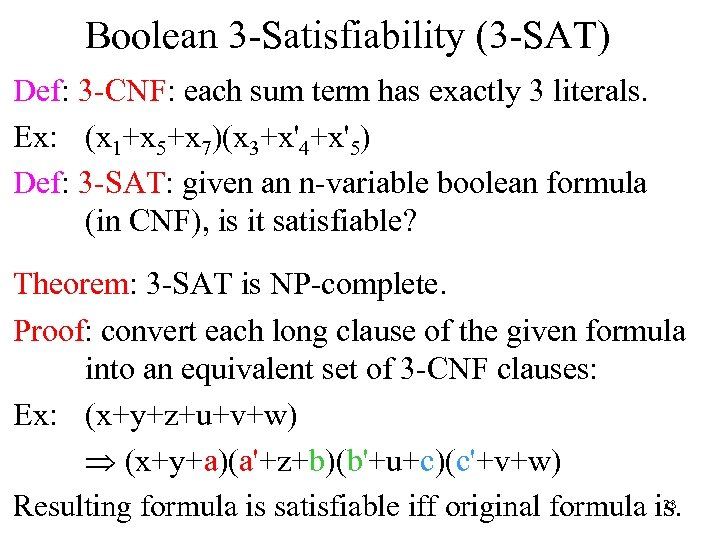Boolean 3 -Satisfiability (3 -SAT) Def: 3 -CNF: each sum term has exactly 3 literals. Ex: (x 1+x 5+x 7)(x 3+x'4+x'5) Def: 3 -SAT: given an n-variable boolean formula (in CNF), is it satisfiable? Theorem: 3 -SAT is NP-complete. Proof: convert each long clause of the given formula into an equivalent set of 3 -CNF clauses: Ex: (x+y+z+u+v+w) Þ (x+y+a)(a'+z+b)(b'+u+c)(c'+v+w) 23 Resulting formula is satisfiable iff original formula is.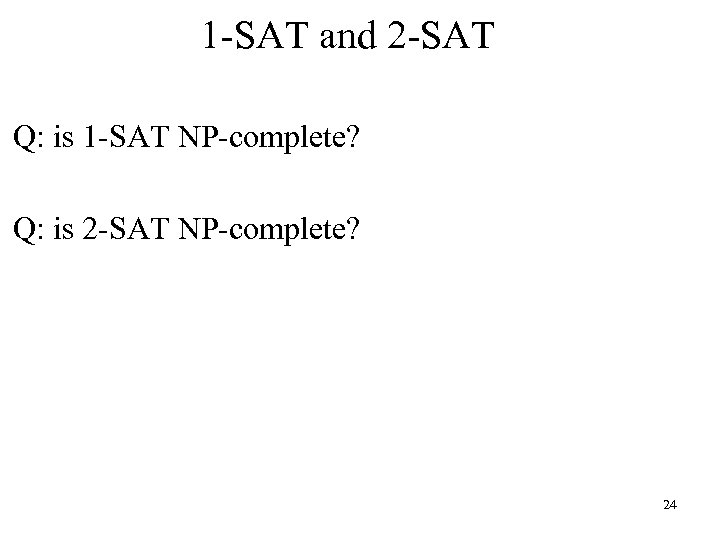1 -SAT and 2 -SAT Q: is 1 -SAT NP-complete? Q: is 2 -SAT NP-complete? 24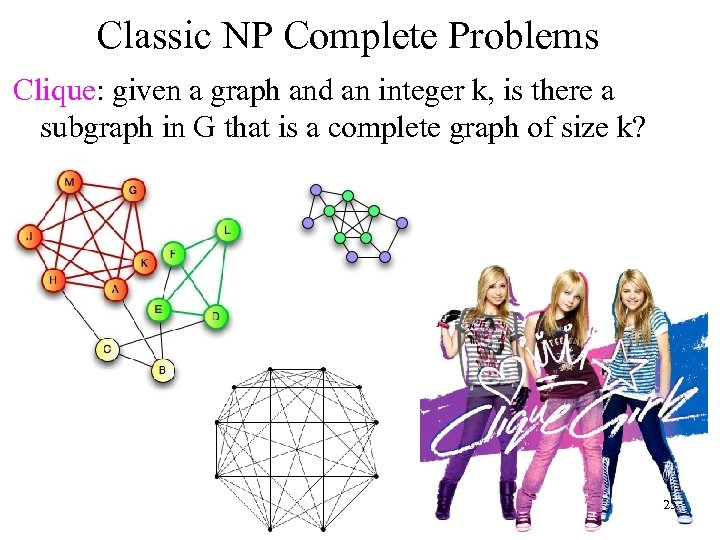Classic NP Complete Problems Clique: given a graph and an integer k, is there a subgraph in G that is a complete graph of size k? 25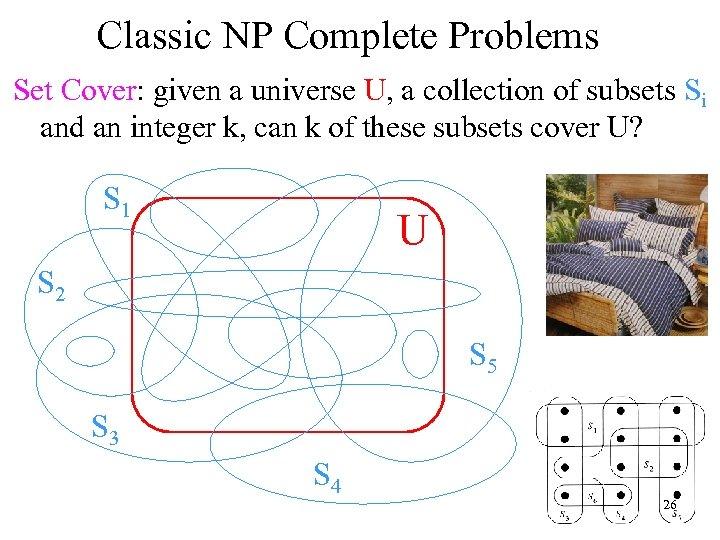Classic NP Complete Problems Set Cover: given a universe U, a collection of subsets Si and an integer k, can k of these subsets cover U? S 1 U S 2 S 5 S 3 S 4 26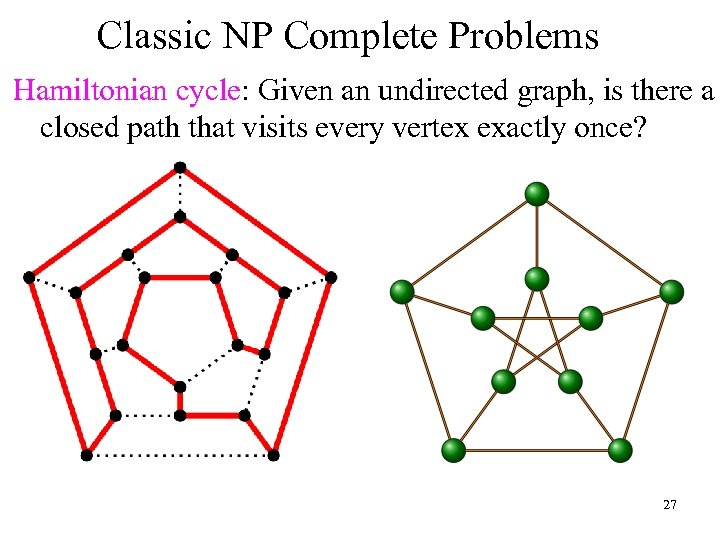Classic NP Complete Problems Hamiltonian cycle: Given an undirected graph, is there a closed path that visits every vertex exactly once? 27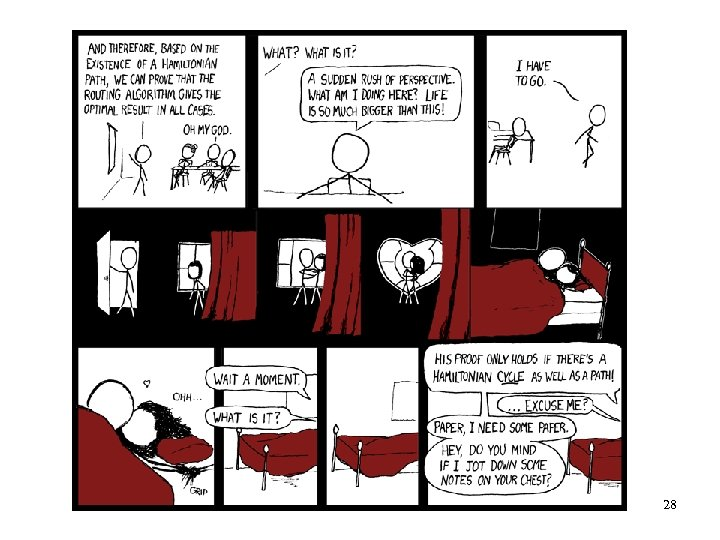28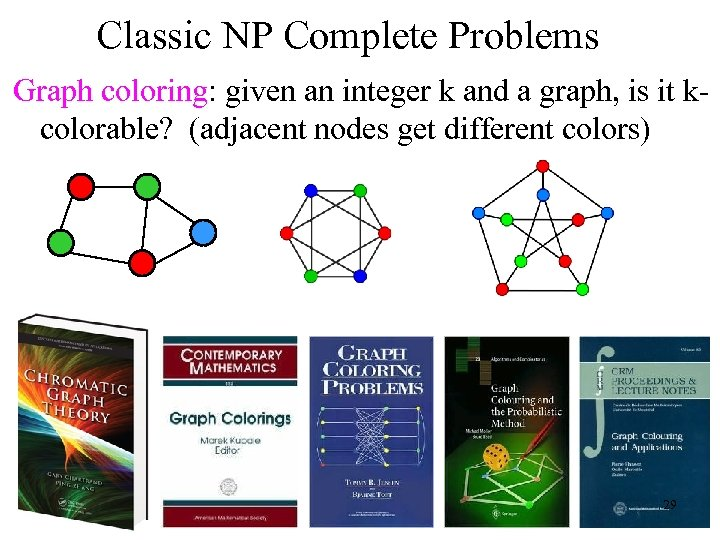Classic NP Complete Problems Graph coloring: given an integer k and a graph, is it kcolorable? (adjacent nodes get different colors) 29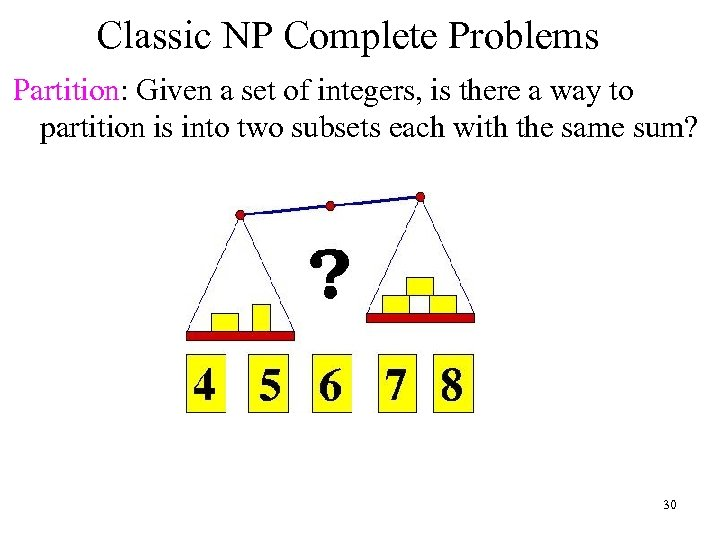Classic NP Complete Problems Partition: Given a set of integers, is there a way to partition is into two subsets each with the same sum? 30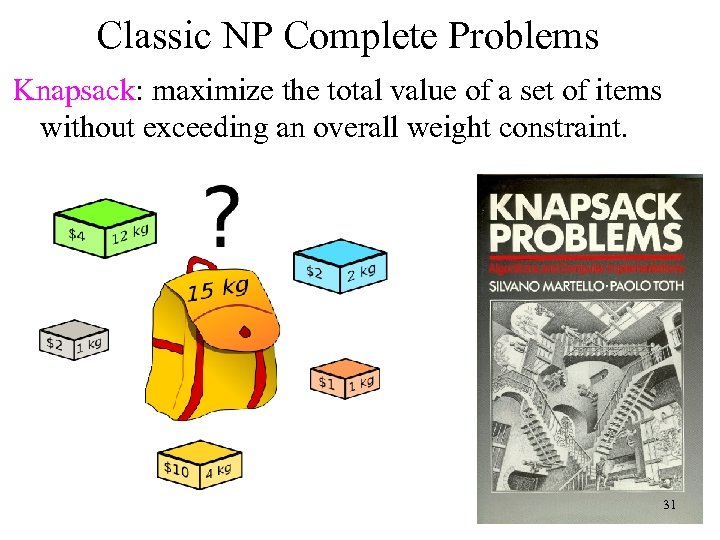Classic NP Complete Problems Knapsack: maximize the total value of a set of items without exceeding an overall weight constraint. 31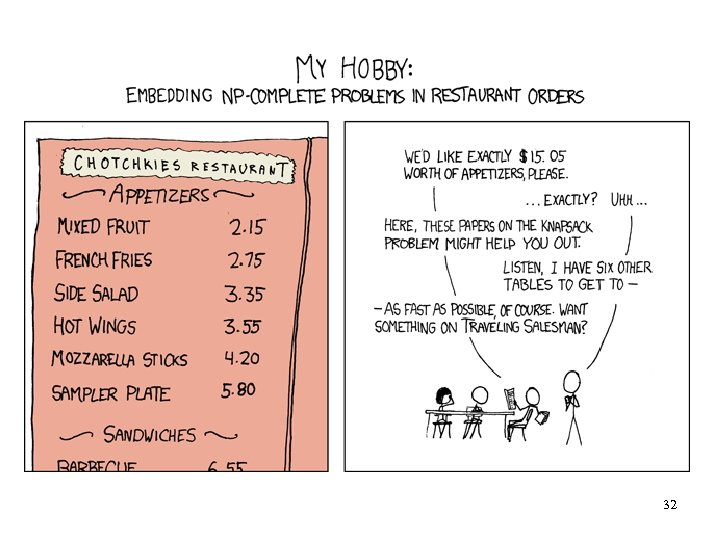32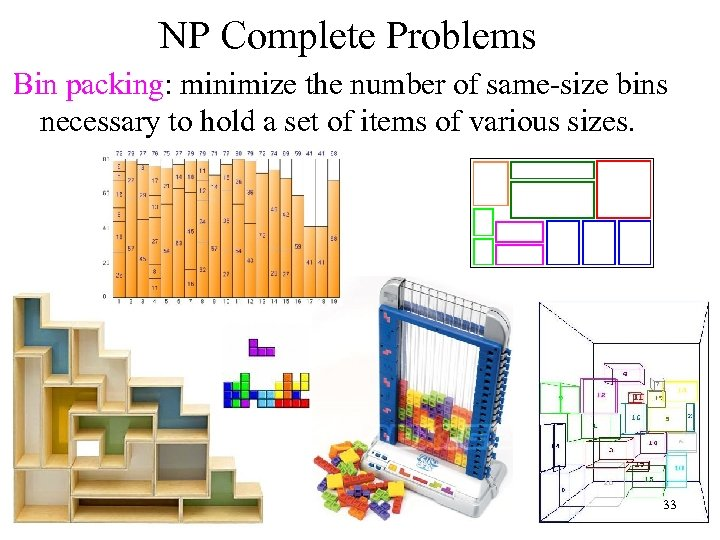NP Complete Problems Bin packing: minimize the number of same-size bins necessary to hold a set of items of various sizes. 33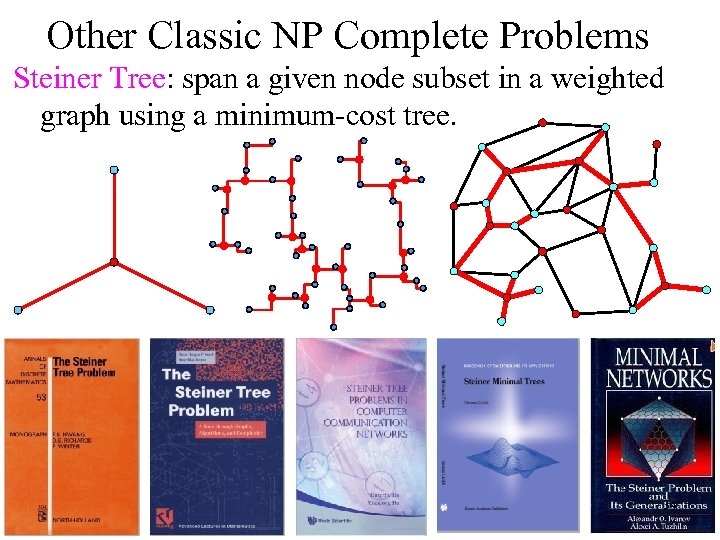Other Classic NP Complete Problems Steiner Tree: span a given node subset in a weighted graph using a minimum-cost tree. 2 34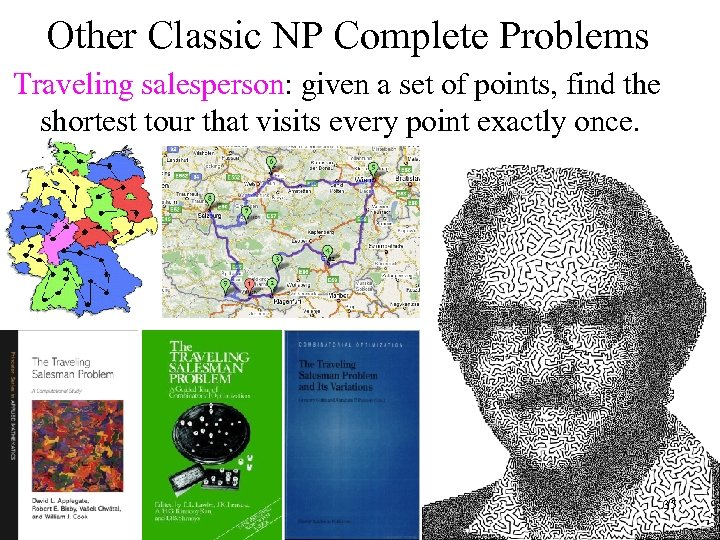Other Classic NP Complete Problems Traveling salesperson: given a set of points, find the shortest tour that visits every point exactly once. 35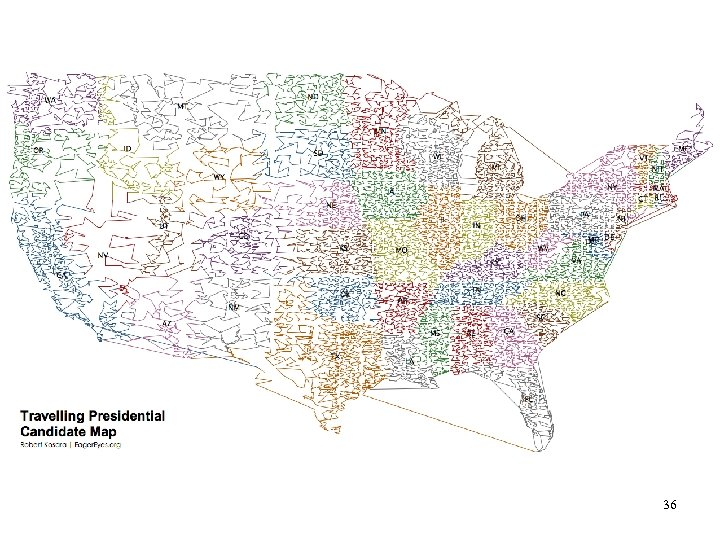3637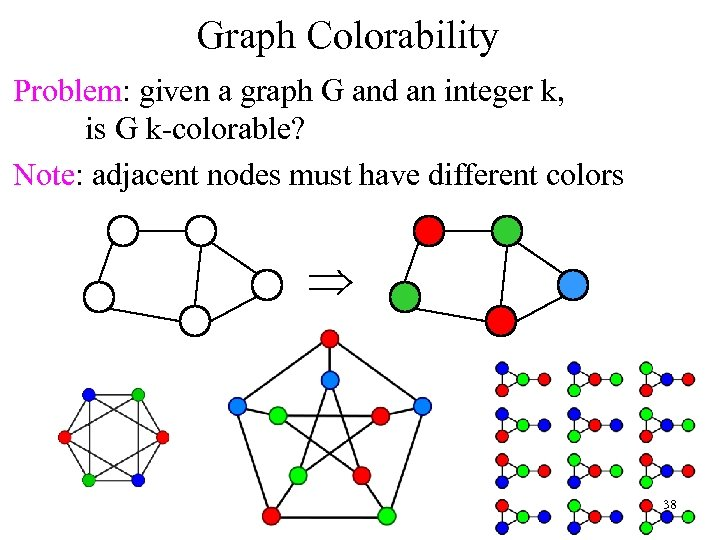Graph Colorability Problem: given a graph G and an integer k, is G k-colorable? Note: adjacent nodes must have different colors Þ 38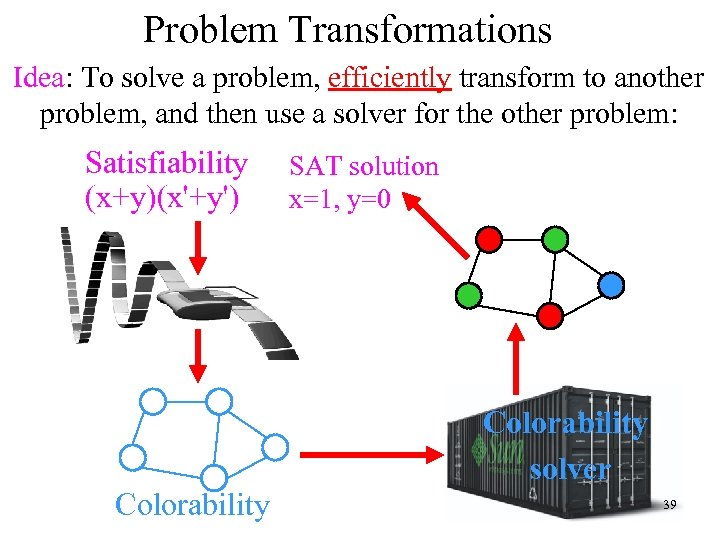Problem Transformations Idea: To solve a problem, efficiently transform to another problem, and then use a solver for the other problem: Satisfiability (x+y)(x'+y') Colorability SAT solution x=1, y=0 Colorability solver 39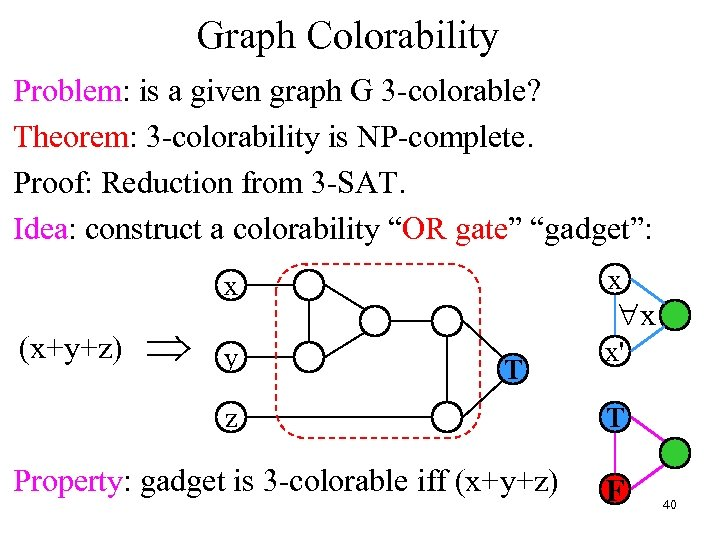Graph Colorability Problem: is a given graph G 3 -colorable? Theorem: 3 -colorability is NP-complete. Proof: Reduction from 3 -SAT. Idea: construct a colorability “OR gate” “gadget”: x (x+y+z) Þ x y x' "x T z Property: gadget is 3 -colorable iff (x+y+z) T F 40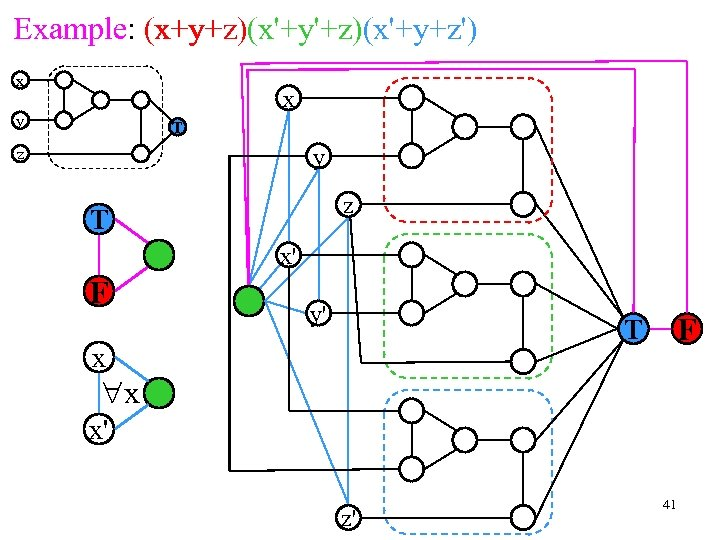x+y+z x'+y'+z x'+y+z' Example: (x+y+z)(x'+y'+z)(x'+y+z') x x y T y z z T x' F y' F T x "x x' z' 41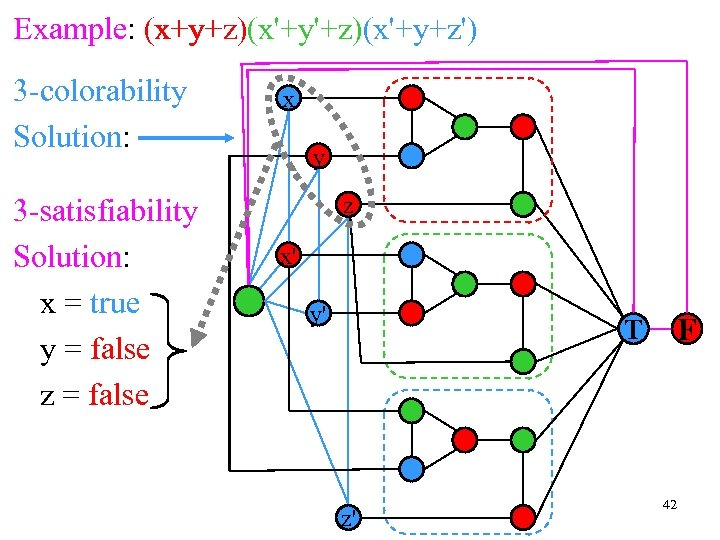x+y+z x'+y'+z x'+y+z' Example: (x+y+z)(x'+y'+z)(x'+y+z') 3 -colorability Solution: 3 -satisfiability Solution: x = true y = false z = false x y z x' y' F T z' 42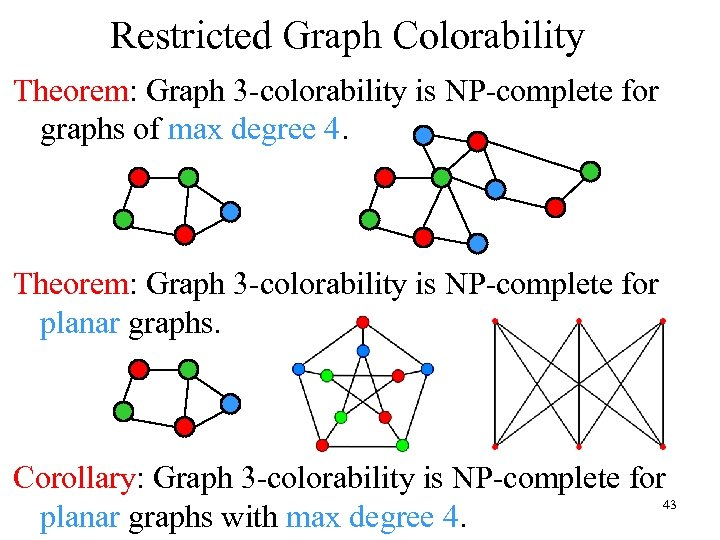Restricted Graph Colorability Theorem: Graph 3 -colorability is NP-complete for graphs of max degree 4. Theorem: Graph 3 -colorability is NP-complete for planar graphs. Corollary: Graph 3 -colorability is NP-complete for 43 planar graphs with max degree 4.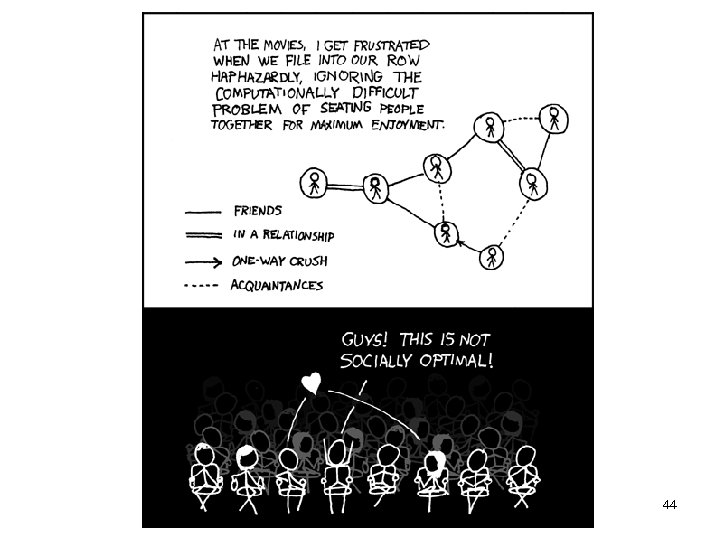44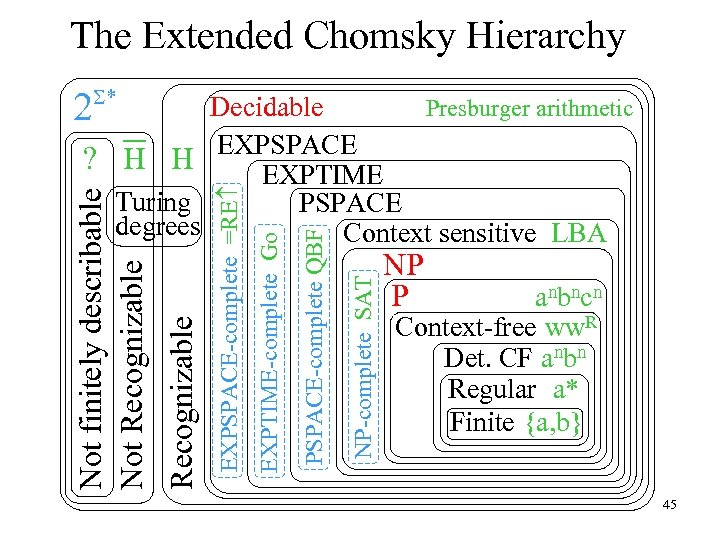The Extended Chomsky Hierarchy 2 S* NP-complete SAT PSPACE-complete QBF EXPTIME-complete Go EXPSPACE-complete =RE Recognizable Not finitely describable Not Recognizable Decidable Presburger arithmetic EXPSPACE ? H H EXPTIME Turing PSPACE degrees Context sensitive LBA NP P anbncn Context-free ww. R Det. CF anbn Regular a* Finite {a, b} 45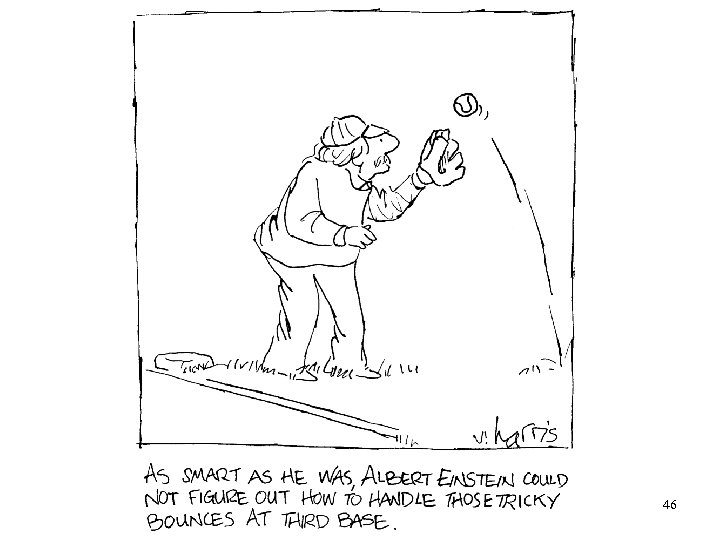46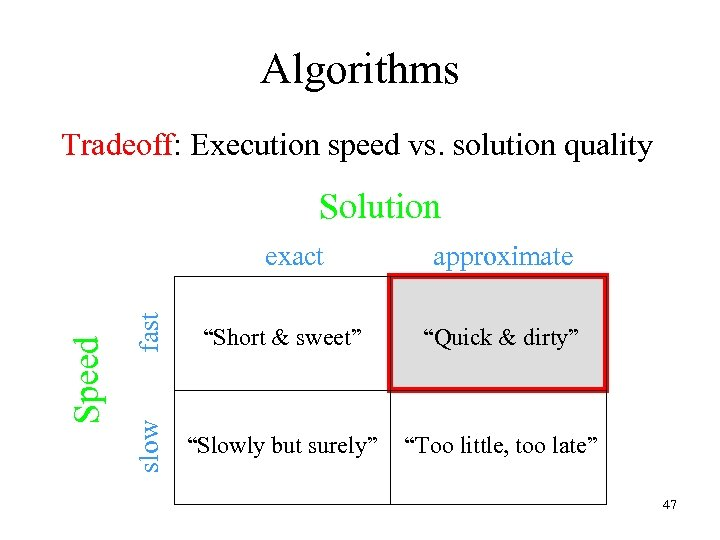Algorithms Tradeoff: Execution speed vs. solution quality Solution fast approximate “Short & sweet” “Quick & dirty” slow Speed exact “Slowly but surely” “Too little, too late” 47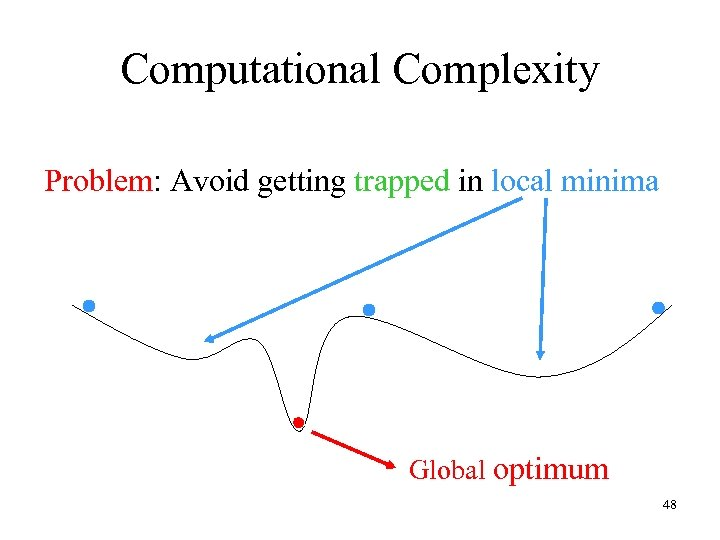Computational Complexity Problem: Avoid getting trapped in local minima Global optimum 48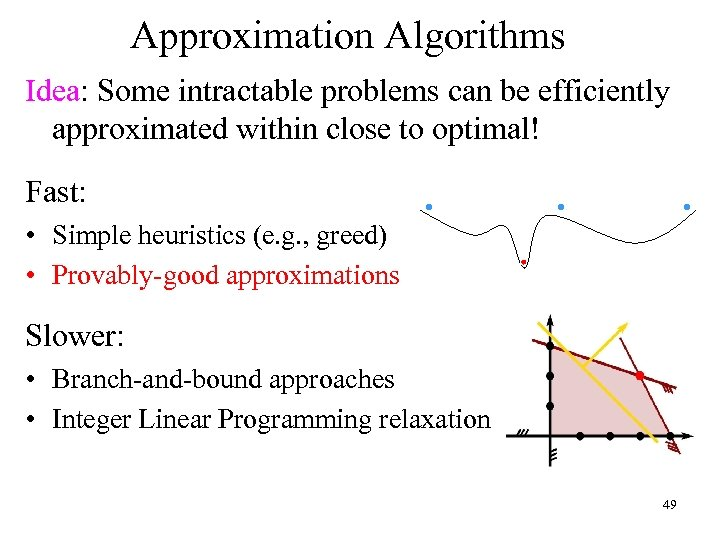Approximation Algorithms Idea: Some intractable problems can be efficiently approximated within close to optimal! Fast: • Simple heuristics (e. g. , greed) • Provably-good approximations Slower: • Branch-and-bound approaches • Integer Linear Programming relaxation 49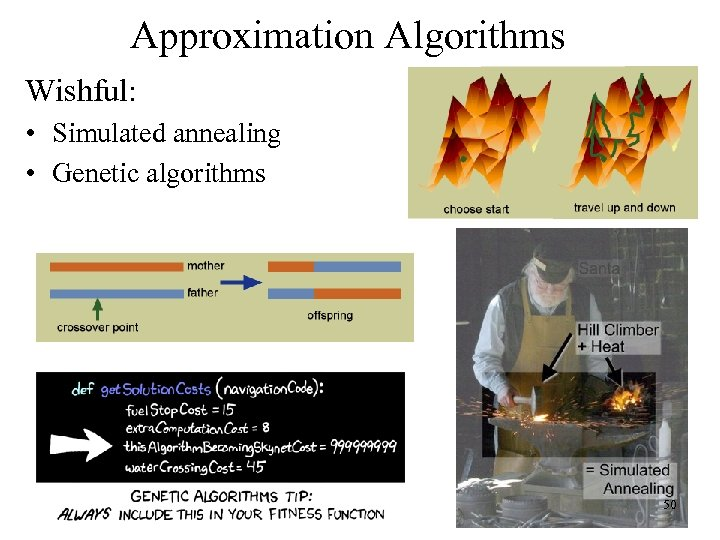Approximation Algorithms Wishful: • Simulated annealing • Genetic algorithms 50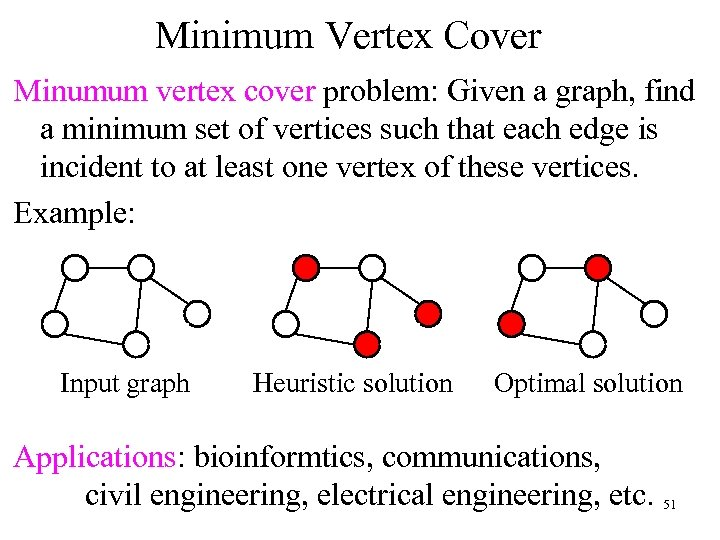Minimum Vertex Cover Minumum vertex cover problem: Given a graph, find a minimum set of vertices such that each edge is incident to at least one vertex of these vertices. Example: Input graph Heuristic solution Optimal solution Applications: bioinformtics, communications, civil engineering, electrical engineering, etc. 51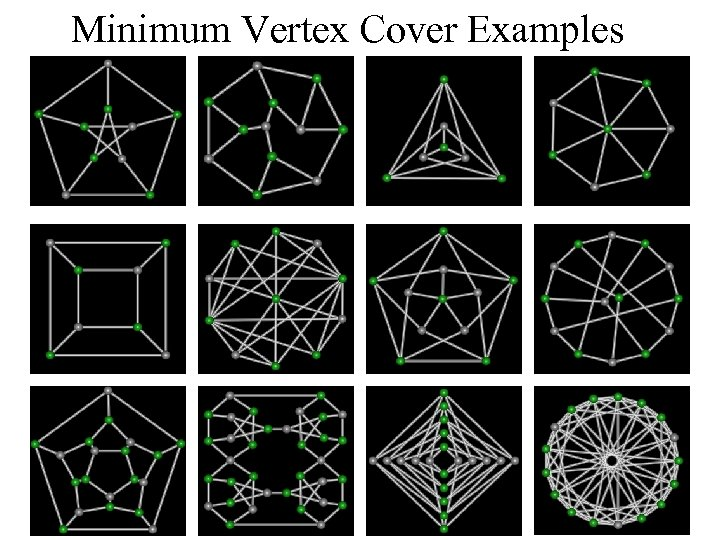Minimum Vertex Cover Examples 52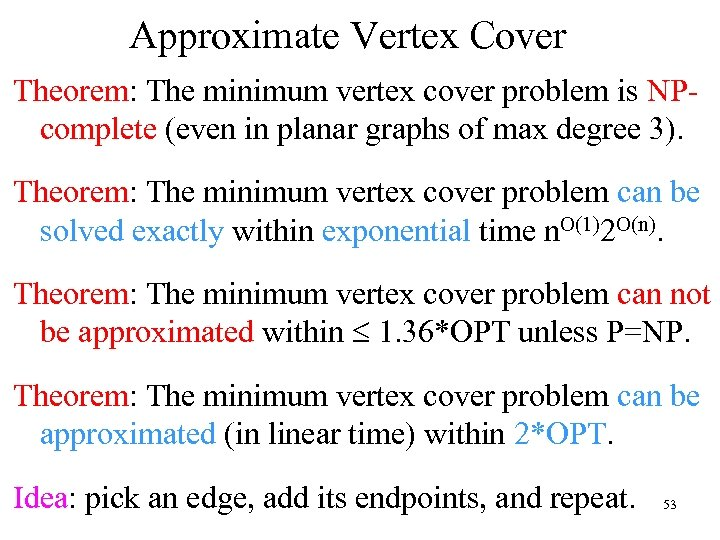Approximate Vertex Cover Theorem: The minimum vertex cover problem is NPcomplete (even in planar graphs of max degree 3). Theorem: The minimum vertex cover problem can be solved exactly within exponential time n. O(1)2 O(n). Theorem: The minimum vertex cover problem can not be approximated within £ 1. 36*OPT unless P=NP. Theorem: The minimum vertex cover problem can be approximated (in linear time) within 2*OPT. Idea: pick an edge, add its endpoints, and repeat. 53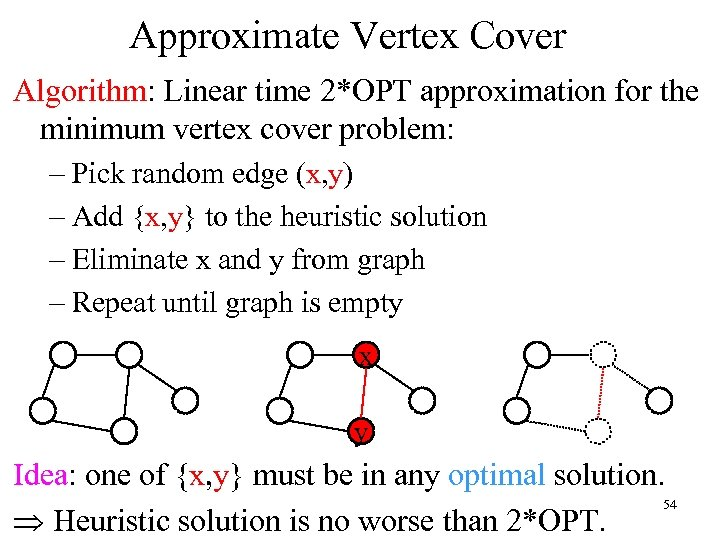Approximate Vertex Cover Algorithm: Linear time 2*OPT approximation for the minimum vertex cover problem: – Pick random edge (x, y) – Add {x, y} to the heuristic solution – Eliminate x and y from graph – Repeat until graph is empty x y Idea: one of {x, y} must be in any optimal solution. 54 Þ Heuristic solution is no worse than 2*OPT.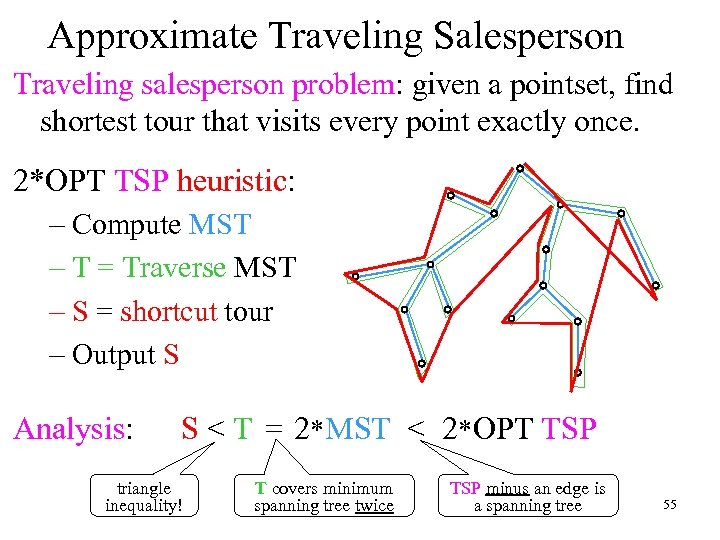Approximate Traveling Salesperson Traveling salesperson problem: given a pointset, find shortest tour that visits every point exactly once. 2*OPT TSP heuristic: – Compute MST – T = Traverse MST – S = shortcut tour – Output S Analysis: S < T = 2* MST < 2* OPT TSP triangle inequality! T covers minimum spanning tree twice TSP minus an edge is a spanning tree 55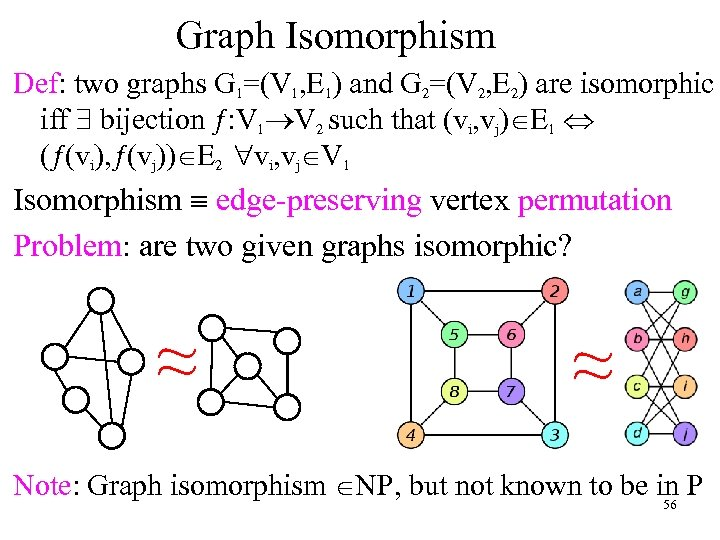Graph Isomorphism Def: two graphs G 1=(V 1, E 1) and G 2=(V 2, E 2) are isomorphic iff \$ bijection ƒ: V 1®V 2 such that (vi, vj)ÎE 1 Û (ƒ(vi), ƒ(vj))ÎE 2 "vi, vjÎV 1 Isomorphism º edge-preserving vertex permutation Problem: are two given graphs isomorphic? ≈ ≈ Note: Graph isomorphism ÎNP, but not known to be in P 56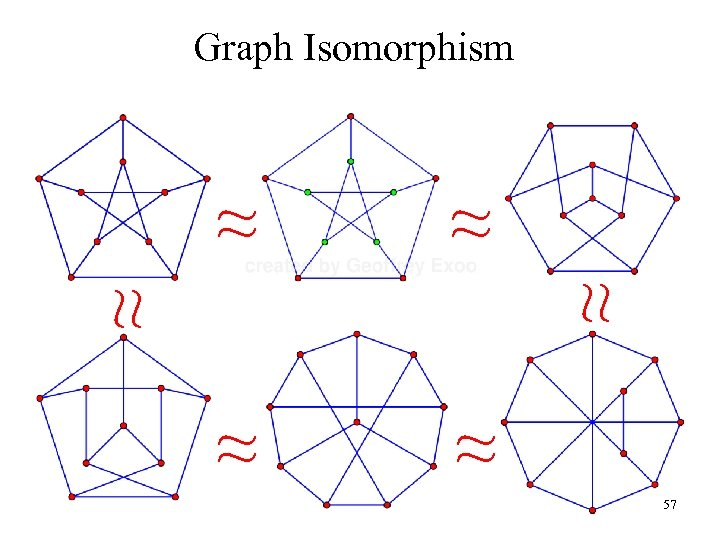Graph Isomorphism ≈ ≈ ≈ 57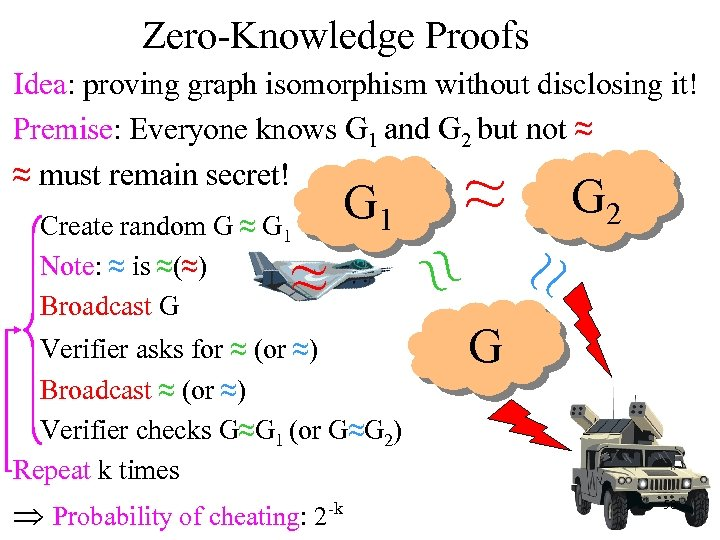Zero-Knowledge Proofs Idea: proving graph isomorphism without disclosing it! Premise: Everyone knows G 1 and G 2 but not ≈ ≈ must remain secret! G 2 G 1 Create random G ≈ G 1 ≈ Verifier asks for ≈ (or ≈) Broadcast ≈ (or ≈) Verifier checks G≈G 1 (or G≈G 2) Repeat k times Þ Probability of cheating: 2 -k ≈ ≈ ≈ Note: ≈ is ≈(≈) Broadcast G G 58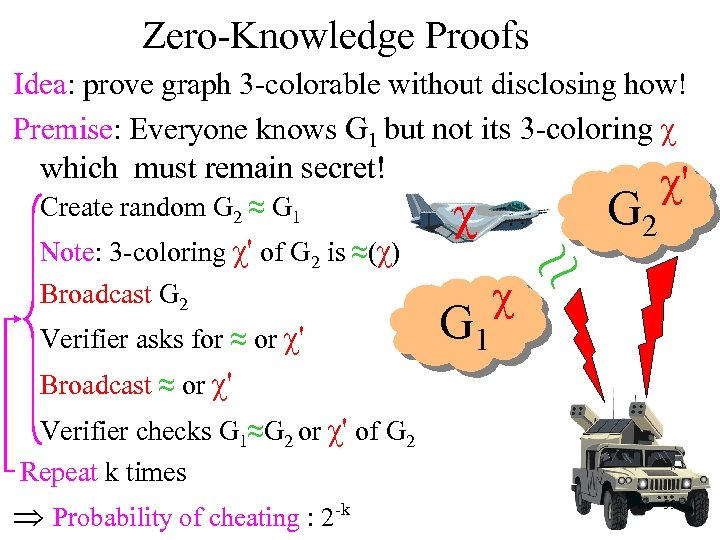Zero-Knowledge Proofs Idea: prove graph 3 -colorable without disclosing how! Premise: Everyone knows G 1 but not its 3 -coloring χ which must remain secret! Create random G 2 ≈ G 1 Note: 3 -coloring χ' of G 2 is ≈(χ) Broadcast G 2 Verifier asks for ≈ or χ' χ G 1 χ ≈ G 2 χ' Broadcast ≈ or χ' Verifier checks G 1≈G 2 or χ' of G 2 Repeat k times Þ Probability of cheating : 2 -k 59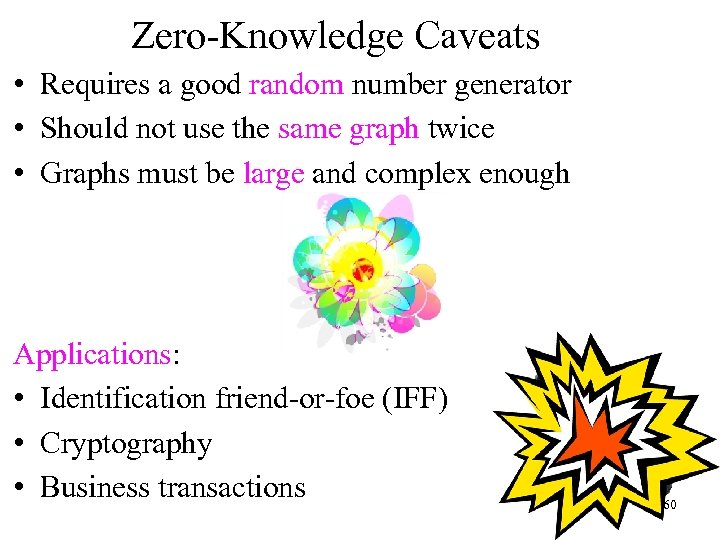Zero-Knowledge Caveats • Requires a good random number generator • Should not use the same graph twice • Graphs must be large and complex enough χ Applications: • Identification friend-or-foe (IFF) • Cryptography • Business transactions 60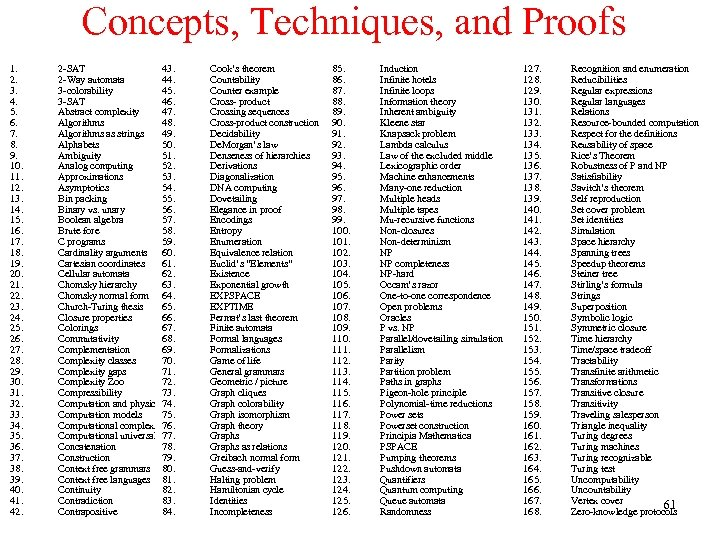Concepts, Techniques, and Proofs 1. 2. 3. 4. 5. 6. 7. 8. 9. 10. 11. 12. 13. 14. 15. 16. 17. 18. 19. 20. 21. 22. 23. 24. 25. 26. 27. 28. 29. 30. 31. 32. 33. 34. 35. 36. 37. 38. 39. 40. 41. 42. 2 -SAT 43. 2 -Way automata 44. 3 -colorability 45. 3 -SAT 46. Abstract complexity 47. Algorithms 48. Algorithms as strings 49. Alphabets 50. Ambiguity 51. Analog computing 52. Approximations 53. Asymptotics 54. Bin packing 55. Binary vs. unary 56. Boolean algebra 57. Brute fore 58. C programs 59. Cardinality arguments 60. Cartesian coordinates 61. Cellular automata 62. Chomsky hierarchy 63. Chomsky normal form 64. Church-Turing thesis 65. Closure properties 66. Colorings 67. Commutativity 68. Complementation 69. Complexity classes 70. Complexity gaps 71. Complexity Zoo 72. Compressibility 73. Computation and physics 74. Computation models 75. Computational complexity 76. Computational universality 77. Concatenation 78. Construction 79. Context free grammars 80. Context free languages 81. Continuity 82. Contradiction 83. Contrapositive 84. Cook’s theorem Countability Counter example Cross- product Crossing sequences Cross-product construction Decidability De. Morgan’s law Denseness of hierarchies Derivations Diagonalization DNA computing Dovetailing Elegance in proof Encodings Entropy Enumeration Equivalence relation Euclid’s “Elements” Existence Exponential growth EXPSPACE EXPTIME Fermat’s last theorem Finite automata Formal languages Formalizations Game of life General grammars Geometric / picture Graph cliques Graph colorability Graph isomorphism Graph theory Graphs as relations Greibach normal form Guess-and-verify Halting problem Hamiltonian cycle Identities Incompleteness 85. 86. 87. 88. 89. 90. 91. 92. 93. 94. 95. 96. 97. 98. 99. 100. 101. 102. 103. 104. 105. 106. 107. 108. 109. 110. 111. 112. 113. 114. 115. 116. 117. 118. 119. 120. 121. 122. 123. 124. 125. 126. Induction Infinite hotels Infinite loops Information theory Inherent ambiguity Kleene star Knapsack problem Lambda calculus Law of the excluded middle Lexicographic order Machine enhancements Many-one reduction Multiple heads Multiple tapes Mu-recursive functions Non-closures Non-determinism NP NP completeness NP-hard Occam’s razor One-to-one correspondence Open problems Oracles P vs. NP Parallel/dovetailing simulation Parallelism Parity Partition problem Paths in graphs Pigeon-hole principle Polynomial-time reductions Power sets Powerset construction Principia Mathematica PSPACE Pumping theorems Pushdown automata Quantifiers Quantum computing Queue automata Randomness 127. 128. 129. 130. 131. 132. 133. 134. 135. 136. 137. 138. 139. 140. 141. 142. 143. 144. 145. 146. 147. 148. 149. 150. 151. 152. 153. 154. 155. 156. 157. 158. 159. 160. 161. 162. 163. 164. 165. 166. 167. 168. Recognition and enumeration Reducibilities Regular expressions Regular languages Relations Resource-bounded computation Respect for the definitions Reusability of space Rice’s Theorem Robustness of P and NP Satisfiability Savitch’s theorem Self reproduction Set cover problem Set identities Simulation Space hierarchy Spanning trees Speedup theorems Steiner tree Stirling’s formula Strings Superposition Symbolic logic Symmetric closure Time hierarchy Time/space tradeoff Tractability Transfinite arithmetic Transformations Transitive closure Transitivity Traveling salesperson Triangle inequality Turing degrees Turing machines Turing recognizable Turing test Uncomputability Uncountability Vertex cover 61 Zero-knowledge protocols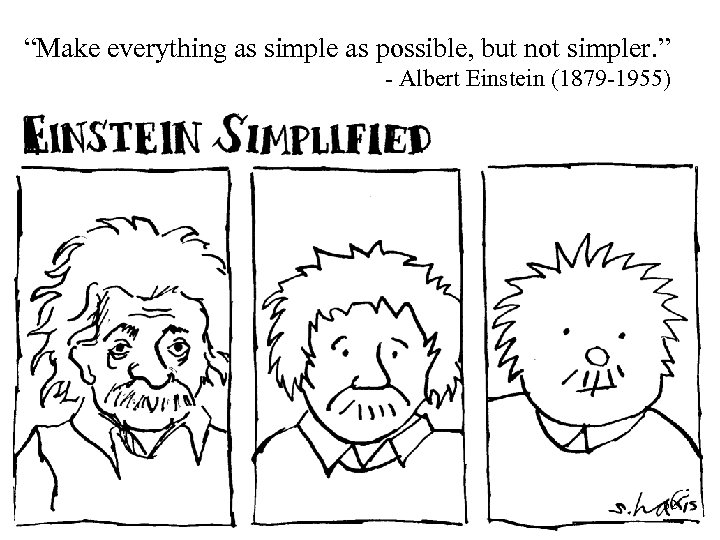“Make everything as simple as possible, but not simpler. ” - Albert Einstein (1879 -1955) 62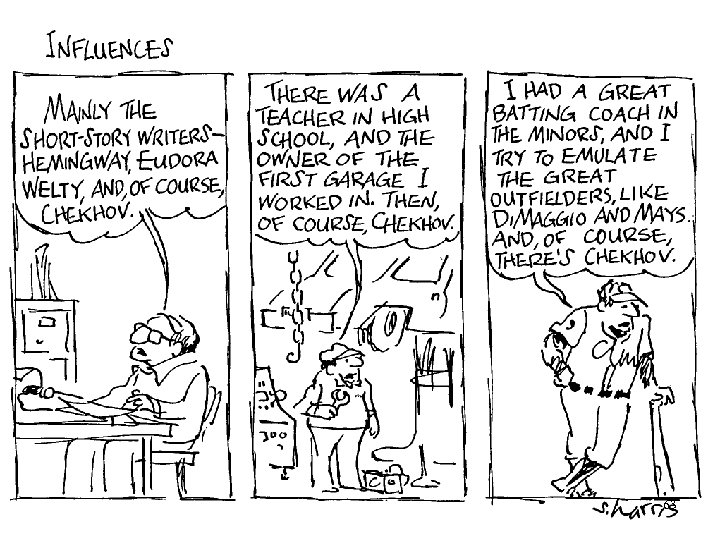63